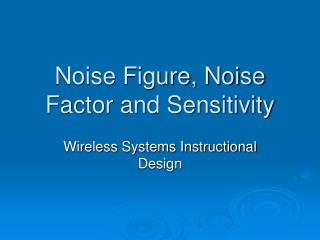DownloadDownload PresentationNoise Figure, Noise Factor and Sensitivity

# Noise Figure, Noise Factor and Sensitivity

Télécharger la présentation## Noise Figure, Noise Factor and Sensitivity

- - - - - - - - - - - - - - - - - - - - - - - - - - - E N D - - - - - - - - - - - - - - - - - - - - - - - - - - -
##### Presentation Transcript

1. Noise Figure, Noise Factor and Sensitivity Wireless Systems Instructional Design

2. Noise • “Any unwanted input” • Limits systems ability to process weak signals • Sources: • Random noise in resistors and transistors • Mixer noise • Undesired cross-coupling noise • Power supply noise • Dynamic range – capability of detecting weak signals in presence of large-amplitude signals

3. Noise (contd.) • “noisiness” of the signal measure = signal-to-noise ratio (frequency dependant) • Random noise • External • Atmospheric • Interstellar • Receiver internal • Thermal • Flicker noise (low frequency) • Shot noise

4. “Sky” Noise

5. Noise factor • IEEE Standards: “The noise factor, at a specified input frequency, is defined as the ratio of (1) the total noise power per unit bandwidth available at the output port when noise temperature of the input termination is standard (290 K) to (2) that portion of (1) engendered at the input frequency by the input termination.”

6. Noise factor (cont.) • It is a measure of the degradation of SNR due to the noise added - • Implies that SNR gets worse as we process the signal • Spot noise factor • The answer is the bandwidth

7. Noise factor (cont.) • Quantitative measure of receiver performance wrt noise for a given bandwidth • Noise figure • Typically 8-10 db for modern receivers • Multistage (cascaded) system

8. Sensitivity • Minimum detectable input signal level for a given output SNR (also called noise floor) • Not necessarily related to required output SNR • Example

9. Link Budget • “Quick and dirty” way of estimating RF link performance • Prx,Ptx – received and transmitted power (dB) • Grx,Gtx – antenna gain (dBi) • L – path loss • Amisc – miscellaneous attenuation

10. Link Budget (cont.) • Path loss (Friis formula): • L = 40 dB + 20log(d) @ 2.4 GHz • L = 48 dB + 20log(d) @ 5.7 GHz • Transmit power: • 15 – 20 dBm (30 – 100 mW) • Antenna gain: given in decibels over an isotropic antenna (dBi) • 0dBi (isotropic), 8 dBi (biquad), 15 dBi (helix), 24 dBi (parabolic)